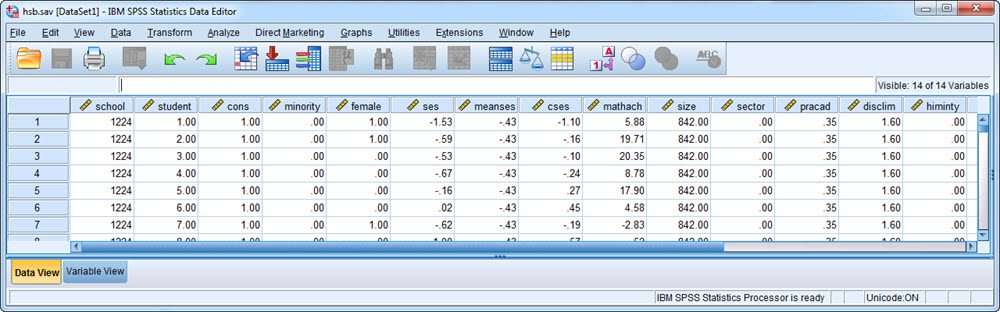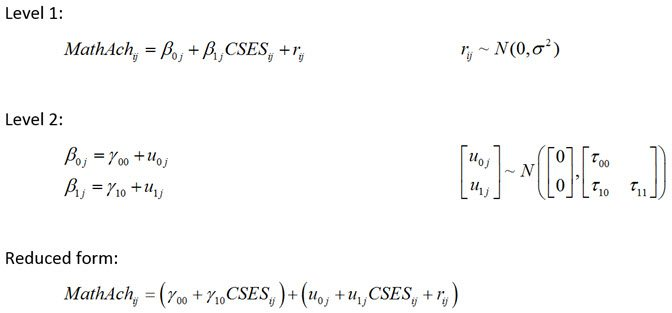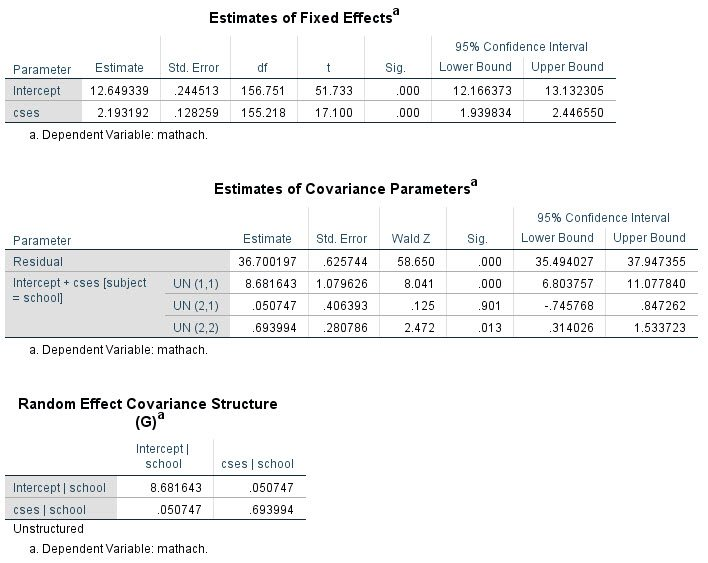Generating and Saving Random Effect Estimates in SPSS (Version 25+)

Note: As of version 25, SPSS includes an option to print the random effect estimates to the output window. The Output Management System (OMS) can then be used to save these estimates to a data file. The instructions below illustrate how to use these options. On a separate page, we provide information on how to generate and save random effect estimates in earlier versions of SPSS that do not have this option.

Like SAS, Stata, R, and many other statistical software programs, SPSS provides the ability to fit multilevel models (also known as hierarchical linear models, mixed-effects models, random effects models, and variance component models). Saving estimates of the random effects to a data file can, however, be a bit tricky in SPSS. These estimates are useful for a variety of purposes, for instance to conduct model diagnostics. Plots involving these estimates can help to evaluate whether the random effects are plausibly normally distributed, whether there are extreme values, and whether predictors may have omitted nonlinear effects. Sometimes it is also of interest to rank cases by the estimated values of the random effects, or to use the random effect estimates for the purpose of plotting individual trajectories (particularly in the presence of covariates). To perform these analyses, we need to both produce the random effect estimates and save them to a data file. As of version 25, this process has become much easier, as illustrated below (if you have an earlier version, see the alternate instructions). Note that, for any given analysis, minor changes to the syntax will be required to reflect the data and model at hand.

You may wish to right-click these links and choose "Save As" to preserve formatting.

Example

To illustrate, we will implement the syntax file for the High School and Beyond example used in the textbook by Raudenbush and Bryk (2002) and which figures in software tutorials by Peugh and Enders (2005) and Singer (1998). In this example, there are 7185 students nested within 160 high schools. Within SPSS, the data looks like this:The model considered here evaluates the within-school effect of socioeconomic status on math achievement and whether this effect varies over schools. In equation form, the model isNote that the level-1 units are students within schools, the level-2 units are schools, i indexes the student, j indexes the school, MathAch is student math achievement, and CSES is a within-school centered measure of the student's socioeconomic status. The level-1 residuals are denoted r with variance sigma-squared, and the Level-2 random effects are denoted with u's and their variances and covariances are represented by tau's.

To fit this model in SPSS, we would use the following syntax (see Peugh and Enders, 2005, for a detailed explanation of most of these commands):

MIXED mathach WITH cses
/FIXED=cses
/RANDOM=INTERCEPT cses | SUBJECT(school) COVTYPE(UN) SOLUTION
/PRINT=SOLUTION TESTCOV G.

The novel syntax option within SPSS v. 25+ is the SOLUTION option on the /RANDOM subcommand.  This tells SPSS to generate a table of output containing the random effect estimates for the schools.

The usual output from this model is shown here:The new output with the random effect estimates for each Level 2 unit produced by the SOLUTION option on the RANDOM subcommand is shown here:(Note: This table was reformatted to show four numbers after the decimal; in the original output, each value was rounded to the nearest whole number)

The numerical values in the prediction column are the empirical Bayes’ estimates of the random effects, with one entry per cluster (here school). Thus, for School 1224, the random intercept and slope estimates (u0 and u1) are predicted to be -2.6780 and .0698.  These numbers indicate that, relative to the average school, School 1224 shows lower average math achievement and a slightly stronger relationship between SES and math achievement (these interpretations follow from the group-mean centering of CSES).

To be most useful, we need to capture these random effect estimates in a data file.  The easiest way to do this is by using the Output Management System (OMS).

OMS is a handy feature of SPSS that can be used, among other things, to take any output table and save it to a data file. In effect, you can take any set of syntax commands and put an OMS "wrapper" around it to generate data from the output. We can break this down into three steps.

Step 1: Use FILE HANDLE to declare the directory/folder where you will store the new data file:

FILE HANDLE directory
/NAME='C:UsersdbauerDesktop'.

This step is not absolutely necessary but if offers the convenience of only needing to give the full reference for this location once.

Step 2: Write the front end of the OMS wrapper:

OMS SELECT TABLES
/DESTINATION FORMAT = sav OUTFILE="directoryREs.sav"
/IF COMMANDS = ['mixed'] subtypes=['empirical best linear unbiased predictions'].

This tells SPSS that I want to take the table of output labeled 'empirical best linear unbiased predictions (indicated in the subtypes= option) that will be generated by the mixed command (commands= option) and output it as an SPSS data file named REs.sav in the directory that I declared above.  How did I know the correct name for the table? I simply looked at the title SPSS gave the output when I ran the model previously.

Step 3: Specify the model with the MIXED command using the SOLUTION option in the /RANDOM subcommand (same as before) and then type OMSEND as the backend of the wrapper:

MIXED mathach WITH cses
/FIXED=cses
/RANDOM=INTERCEPT cses | SUBJECT(school) COVTYPE(UN) SOLUTION
/PRINT=SOLUTION TESTCOV G.

OMSEND.

Running this, we'll simply see the same output as before, but now we've also created the REs.sav data file with the random effect estimates. We can pull this into an active data window as follows:

GET FILE "directoryREs.sav".
DATASET NAME REs WINDOW=FRONT.

The data set is shown here:As can be seen, the values here from Var1 to UpperBound perfectly reproduce the information from the output table with the random effect estimates.

This data file may already be suited to your purposes but, more often than not, I find that some rearrangement is needed.  In particular, I like to cut this down to just the information of interest (the ID variable and the random effect estimates), rename the variables to be more intuitive, and re-arrange it to one line per cluster with u0 and u1 as separate variables.

The code below deletes the extraneous variables and renames Var1 to ID and Var2 to effect.  If the ID variable in your original data has a different name, use that instead.  Also, the final line here converts ID to a numeric variable.  If your ID variable isn't numeric then just omit this line.

DELETE VARIABLES Command_ Subtype_ Label_ std.error df sig lowerbound upperbound.
RENAME VARIABLES (Var1 = ID) (Var2 = effect).

ALTER TYPE ID (f8.0).

The result of running these commands is a data set with just three variables, as shown here:Now we can re-arrange this to one line per cluster (school) and rename the random effect estimates from Intercept and cses to u0 and u1 with the following commands:

CASESTOVARS
/ID=ID
/INDEX=effect
/GROUPBY=VARIABLE.

RENAME VARIABLES (intercept = u0) (cses = u1).
VARIABLE LABELS u0 'u0' u1 'u1'.

The final result is shown here:Notice that we now have 160 lines of data corresponding to our 160 schools, each school is indicated by its ID number, and we have the random effect estimates as the variables u0 and u1.

From here we can do a variety of useful things, such as examine histograms of u0 and u1, a scatterplot of u0 with u1, or match-merge this file with school-level data to plot u0 and u1 against variables in or out of the fitted model.  These can be important diagnostics to conduct when evaluating the assumptions of the model.

References

Peugh, J.L. & Enders, C.K. (2005). Using the SPSS MIXED procedure to fit cross-sectional and longitudinal multilevel models. Educational and Psychological Measurement, 65, , 717-741.

Raudenbush, S. W., & Bryk, A. S. (2002). Hierarchical linear models: Applications and data analysis methods (2nd ed.). Newbury Park, CA: Sage.

Singer, J. D. (1998). Using SAS PROC MIXED to fit multilevel models, hierarchical models, and individual growth models. Journal of Educational and Behavioral Statistics, 24, 323-355